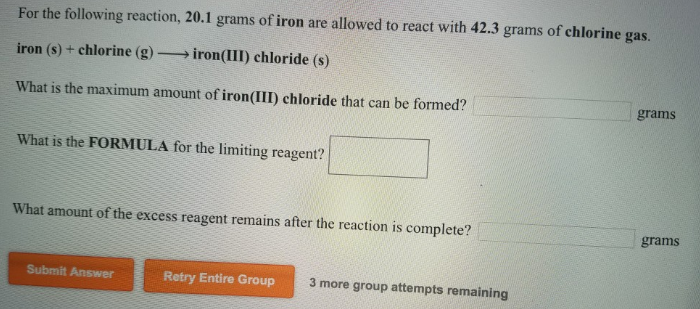# Problem: For the following reaction, 20.1 grams of iron are allowed to react with 42.3 grams of chlorine gas.iron (s) + chlorine (g) → iron(III) chloride (s) What is the maximum amount of iron(III) chloride that can be formed? What is the FORMULA for the limiting reagent? What amount of the excess reagent remains after the reaction is complete?

###### FREE Expert Solution
79% (488 ratings)###### Problem Details

For the following reaction, 20.1 grams of iron are allowed to react with 42.3 grams of chlorine gas.

iron (s) + chlorine (g) → iron(III) chloride (s)

What is the maximum amount of iron(III) chloride that can be formed?

What is the FORMULA for the limiting reagent?

What amount of the excess reagent remains after the reaction is complete?